# JPWO2015190488A1 - Shift register circuit and display device including the same - Google Patents

## Info

Publication number
JPWO2015190488A1
JPWO2015190488A1 JP2015066621A JP2016527828A JPWO2015190488A1 JP WO2015190488 A1 JPWO2015190488 A1 JP WO2015190488A1 JP 2015066621 A JP2015066621 A JP 2015066621A JP 2016527828 A JP2016527828 A JP 2016527828A JP WO2015190488 A1 JPWO2015190488 A1 JP WO2015190488A1
Authority
JP
Japan
Prior art keywords
tft
gate
gate line
potential
drive circuit
Prior art date
Legal status (The legal status is an assumption and is not a legal conclusion. Google has not performed a legal analysis and makes no representation as to the accuracy of the status listed.)
Granted
Application number
JP2015066621A
Other languages
Japanese (ja)
Inventor

Original Assignee
シャープ株式会社
Priority date (The priority date is an assumption and is not a legal conclusion. Google has not performed a legal analysis and makes no representation as to the accuracy of the date listed.)
Filing date
Publication date
Priority to JP2014122391 priority Critical
Priority to JP2014122391 priority
Application filed by シャープ株式会社 filed Critical シャープ株式会社
Priority to PCT/JP2015/066621 priority patent/WO2015190488A1/en
Publication of JPWO2015190488A1 publication Critical patent/JPWO2015190488A1/en
Granted legal-status Critical Current

• 239000003990 capacitor Substances 0.000 claims abstract description 38
• 239000000758 substrates Substances 0.000 claims description 41
• 239000011159 matrix materials Substances 0.000 claims description 33
• 230000000875 corresponding Effects 0.000 claims description 29
• 239000004973 liquid crystal related substances Substances 0.000 claims description 7
• 239000010410 layers Substances 0.000 claims description 6
• 238000000034 methods Methods 0.000 description 1

## Images

••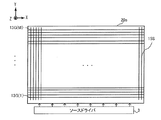•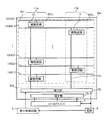••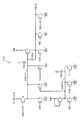•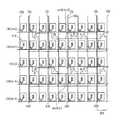••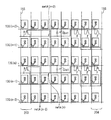••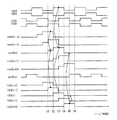•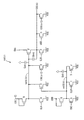•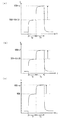••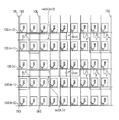•••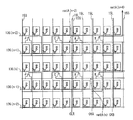••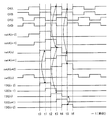•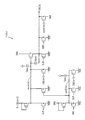•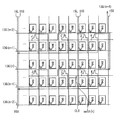•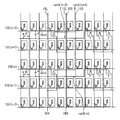•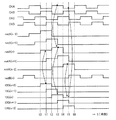•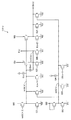•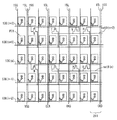••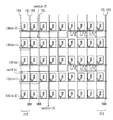•••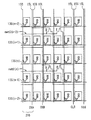•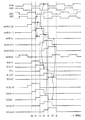•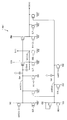•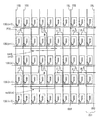•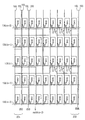•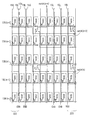•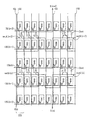••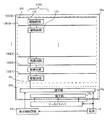•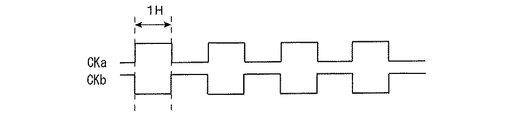•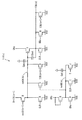•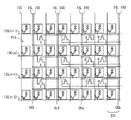•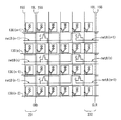•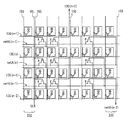••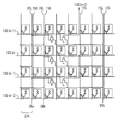•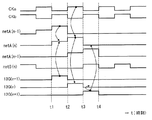•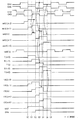## Classifications

• GPHYSICS
• G09EDUCATION; CRYPTOGRAPHY; DISPLAY; ADVERTISING; SEALS
• G09GARRANGEMENTS OR CIRCUITS FOR CONTROL OF INDICATING DEVICES USING STATIC MEANS TO PRESENT VARIABLE INFORMATION
• G09G3/00Control arrangements or circuits, of interest only in connection with visual indicators other than cathode-ray tubes
• G09G3/20Control arrangements or circuits, of interest only in connection with visual indicators other than cathode-ray tubes for presentation of an assembly of a number of characters, e.g. a page, by composing the assembly by combination of individual elements arranged in a matrix no fixed position being assigned to or needed to be assigned to the individual characters or partial characters
• G09G3/34Control arrangements or circuits, of interest only in connection with visual indicators other than cathode-ray tubes for presentation of an assembly of a number of characters, e.g. a page, by composing the assembly by combination of individual elements arranged in a matrix no fixed position being assigned to or needed to be assigned to the individual characters or partial characters by control of light from an independent source
• G09G3/36Control arrangements or circuits, of interest only in connection with visual indicators other than cathode-ray tubes for presentation of an assembly of a number of characters, e.g. a page, by composing the assembly by combination of individual elements arranged in a matrix no fixed position being assigned to or needed to be assigned to the individual characters or partial characters by control of light from an independent source using liquid crystals
• G09G3/3611Control of matrices with row and column drivers
• G09G3/3648Control of matrices with row and column drivers using an active matrix
• GPHYSICS
• G09EDUCATION; CRYPTOGRAPHY; DISPLAY; ADVERTISING; SEALS
• G09GARRANGEMENTS OR CIRCUITS FOR CONTROL OF INDICATING DEVICES USING STATIC MEANS TO PRESENT VARIABLE INFORMATION
• G09G3/00Control arrangements or circuits, of interest only in connection with visual indicators other than cathode-ray tubes
• G09G3/20Control arrangements or circuits, of interest only in connection with visual indicators other than cathode-ray tubes for presentation of an assembly of a number of characters, e.g. a page, by composing the assembly by combination of individual elements arranged in a matrix no fixed position being assigned to or needed to be assigned to the individual characters or partial characters
• G09G3/34Control arrangements or circuits, of interest only in connection with visual indicators other than cathode-ray tubes for presentation of an assembly of a number of characters, e.g. a page, by composing the assembly by combination of individual elements arranged in a matrix no fixed position being assigned to or needed to be assigned to the individual characters or partial characters by control of light from an independent source
• G09G3/36Control arrangements or circuits, of interest only in connection with visual indicators other than cathode-ray tubes for presentation of an assembly of a number of characters, e.g. a page, by composing the assembly by combination of individual elements arranged in a matrix no fixed position being assigned to or needed to be assigned to the individual characters or partial characters by control of light from an independent source using liquid crystals
• G09G3/3611Control of matrices with row and column drivers
• G09G3/3674Details of drivers for scan electrodes
• G09G3/3677Details of drivers for scan electrodes suitable for active matrices only
• GPHYSICS
• G11INFORMATION STORAGE
• G11CSTATIC STORES
• G11C19/00Digital stores in which the information is moved stepwise, e.g. shift register stack stores, push-down stores
• G11C19/28Digital stores in which the information is moved stepwise, e.g. shift register stack stores, push-down stores using semiconductor elements
• GPHYSICS
• G09EDUCATION; CRYPTOGRAPHY; DISPLAY; ADVERTISING; SEALS
• G09GARRANGEMENTS OR CIRCUITS FOR CONTROL OF INDICATING DEVICES USING STATIC MEANS TO PRESENT VARIABLE INFORMATION
• G09G2300/00Aspects of the constitution of display devices
• G09G2300/04Structural and physical details of display devices
• G09G2300/0404Matrix technologies
• G09G2300/0408Integration of the drivers onto the display substrate
• GPHYSICS
• G09EDUCATION; CRYPTOGRAPHY; DISPLAY; ADVERTISING; SEALS
• G09GARRANGEMENTS OR CIRCUITS FOR CONTROL OF INDICATING DEVICES USING STATIC MEANS TO PRESENT VARIABLE INFORMATION
• G09G2300/00Aspects of the constitution of display devices
• G09G2300/04Structural and physical details of display devices
• G09G2300/0421Structural details of the set of electrodes
• G09G2300/0426Layout of electrodes and connections
• GPHYSICS
• G09EDUCATION; CRYPTOGRAPHY; DISPLAY; ADVERTISING; SEALS
• G09GARRANGEMENTS OR CIRCUITS FOR CONTROL OF INDICATING DEVICES USING STATIC MEANS TO PRESENT VARIABLE INFORMATION
• G09G2310/00Command of the display device
• G09G2310/02Addressing, scanning or driving the display screen or processing steps related thereto
• G09G2310/0264Details of driving circuits
• G09G2310/0286Details of a shift registers arranged for use in a driving circuit
• GPHYSICS
• G09EDUCATION; CRYPTOGRAPHY; DISPLAY; ADVERTISING; SEALS
• G09GARRANGEMENTS OR CIRCUITS FOR CONTROL OF INDICATING DEVICES USING STATIC MEANS TO PRESENT VARIABLE INFORMATION
• G09G2310/00Command of the display device
• G09G2310/08Details of timing specific for flat panels, other than clock recovery

## Abstract

Each drive circuit in the shift register includes an output unit, a precharge unit, a booster unit, a gate voltage discharge unit, a gate line discharge unit, and an internal wiring netA. The output unit includes a TFT (F) that outputs a selection voltage to the gate line. The precharge unit includes a TFT (B) that outputs a control voltage for operating the TFT of the output unit. The boosting unit boosts the gate voltage of the TFT of the output unit via the capacitor (Cbst). The gate voltage discharge unit includes a TFT (K) that lowers the gate voltage during a non-selection period of the gate line. The gate line discharge unit includes a TFT (L) that outputs a non-selection voltage to the gate line during a non-selection period of the gate line. The internal wiring is connected to the gate terminal, precharge unit, gate voltage discharge unit, and boost unit of the TFT of the output unit. The gate terminal of at least one TFT in the precharge portion, the gate voltage discharge portion, and the gate line discharge portion is connected to an internal wiring in another drive circuit.

## Description

The present invention relates to a shift register circuit and a display device including the same.

Conventionally, a shift register circuit that sequentially scans a plurality of gate lines provided on an active matrix substrate is known. The shift register circuit includes a drive circuit including an output transistor that switches a gate line to a selected state, a precharge transistor that precharges the gate voltage of the output transistor, and a bootstrap capacitor that boosts the precharge voltage. Prepare for each. A diode-connected transistor is used as the precharge transistor, and the precharge voltage has a voltage value that is lowered by the threshold voltage of the precharge transistor. When the precharge voltage is lowered due to an increase in the threshold voltage due to the deterioration of the transistor, the gate voltage of the output transistor is also lowered, and the operation of the drive circuit becomes unstable. As a result, the operation margin of the shift register circuit is reduced.

Japanese Translation of PCT International Application No. 2008-508654 discloses a shift register circuit that suppresses a decrease in gate voltage of an output transistor depending on a threshold voltage of a precharge transistor. In this shift register circuit, the previous row pulse is input to the drain terminal of the precharge transistor, and a precharge circuit for boosting the gate voltage of the precharge transistor is connected to the gate terminal. In Japanese translation of PCT publication No. 2008-508654, even if the threshold voltage of the precharge transistor fluctuates, the precharge circuit boosts the gate voltage of the precharge transistor and suppresses a decrease in the precharge voltage.

By providing a precharge circuit as in the above-mentioned special table 2008-508654, voltage drop when precharging the gate voltage of the output transistor can be suppressed, but the number of circuit elements in each drive circuit is small. As a result, the circuit scale of the shift register circuit increases.

In addition, when the gate voltage of the output transistor and the discharge of the gate line are insufficient when the gate line is changed to the non-selected state, unintended noise is input to the gate line and the gate line is scanned appropriately. Cannot be performed, and the operation margin of the shift register circuit is lowered.

An object of the present invention is to provide a technique for stably operating each driving circuit in a shift register circuit and improving an operation margin of the shift register circuit.

A shift register circuit according to the present invention is a shift register circuit that switches each of a plurality of gate lines provided on an active matrix substrate to a selected state or a non-selected state, and the shift register circuit is connected to each gate line. A plurality of drive circuits for switching the gate line to a selected state or a non-selected state, each of the drive circuits being connected to one gate line and outputting a selection voltage for switching the one gate line to a selected state An output unit including a switching element, a precharge unit including a switching element that outputs a control voltage for operating the switching element in the output unit, a capacitor, and a switching element that charges the capacitor, Boosting that boosts the gate voltage of the switching element in the output section via a capacitor And a gate voltage discharge unit including a switching element that reduces the gate voltage in a non-selection period for switching the one gate line to a non-selection state, and a non-selection period of the one gate line in the one gate line. A gate line discharge unit including a switching element that outputs a non-selection voltage; and an internal wiring to which the gate terminal of the switching element in the output unit, the precharge unit, the gate voltage discharge unit, and the boost unit are connected. A gate terminal of at least one of the switching elements in the precharge unit, the gate voltage discharge unit, and the gate line discharge unit is connected to the internal wiring in another drive circuit.

According to the configuration of the present invention, each drive circuit in the shift register circuit can be operated stably, and the operation margin of the shift register circuit can be improved.

FIG. 1 is a schematic diagram illustrating a schematic configuration of the liquid crystal display device according to the first embodiment. FIG. 2 is a schematic diagram showing a schematic configuration of the active matrix substrate shown in FIG. FIG. 3 is a schematic diagram showing a schematic configuration of each part connected to the active matrix substrate and the active matrix substrate shown in FIG. FIG. 4 is a diagram illustrating a waveform of the clock signal in the first embodiment. FIG. 5 is a diagram showing an example of an equivalent circuit of the drive circuit shown in FIG. FIG. 6A is a schematic diagram illustrating an arrangement example of elements of the drive circuit illustrated in FIG. 5. FIG. 6B is a schematic diagram illustrating an arrangement example of elements of the drive circuit illustrated in FIG. 5. FIG. 6C is a schematic diagram illustrating an arrangement example of elements of the drive circuit illustrated in FIG. 5. 6D is a schematic diagram illustrating an arrangement example of elements of the drive circuit illustrated in FIG. 5. FIG. 7 is a timing chart when the driving circuit according to the first embodiment drives the gate line. FIG. 8 is a diagram illustrating an example of an equivalent circuit of a conventional drive circuit. FIG. 9 is a diagram for explaining a change in the potential of netA in the conventional drive circuit and the drive circuit in the first embodiment. FIG. 10 is a diagram illustrating an example of an equivalent circuit of the drive circuit in the application example of the first embodiment. FIG. 11 is a schematic diagram showing an arrangement example of elements of the drive circuit shown in FIG. FIG. 12 is a timing chart when the drive circuit in the application example of the first embodiment drives the gate line. FIG. 13 is a diagram showing an equivalent circuit of the drive circuit in the second embodiment. FIG. 14A is a schematic diagram illustrating an arrangement example of some elements of the drive circuit illustrated in FIG. 13. FIG. 14B is a schematic diagram illustrating an arrangement example of some elements of the drive circuit illustrated in FIG. 13. FIG. 15 is a timing chart when the driving circuit according to the second embodiment drives the gate line. FIG. 16 is a diagram illustrating an example of an equivalent circuit of the drive circuit according to the third embodiment. FIG. 17A is a schematic diagram illustrating an arrangement example of some elements of the drive circuit illustrated in FIG. 16. FIG. 17B is a schematic diagram illustrating an arrangement example of some elements of the drive circuit illustrated in FIG. 16. FIG. 18 is a timing chart when the driving circuit according to the third embodiment drives the gate line. FIG. 19 is a diagram illustrating an example of an equivalent circuit of the drive circuit according to the fourth embodiment. FIG. 20A is a schematic diagram illustrating an arrangement example of some elements of the drive circuit illustrated in FIG. 19. FIG. 20B is a schematic diagram illustrating an arrangement example of some elements of the drive circuit illustrated in FIG. 19. FIG. 20C is a schematic diagram illustrating an arrangement example of some elements of the drive circuit illustrated in FIG. 19. FIG. 20D is a schematic diagram illustrating an arrangement example of some elements of the drive circuit illustrated in FIG. 19. FIG. 20E is a schematic diagram illustrating an arrangement example of some elements of the drive circuit illustrated in FIG. 19. FIG. 20F is a schematic diagram illustrating an arrangement example of some elements of the drive circuit illustrated in FIG. 19. FIG. 21 is a timing chart when the drive circuit in the fourth embodiment drives the gate line. FIG. 22 is a diagram illustrating an example of an equivalent circuit of the drive circuit according to the fifth embodiment. FIG. 23A is a schematic diagram illustrating an arrangement example of some elements of the drive circuit illustrated in FIG. 22. FIG. 23B is a schematic diagram illustrating an arrangement example of some elements of the drive circuit illustrated in FIG. 22. FIG. 23C is a schematic diagram illustrating an arrangement example of some elements of the drive circuit illustrated in FIG. 22. FIG. 23D is a schematic diagram illustrating an arrangement example of some elements of the drive circuit illustrated in FIG. 22. FIG. 24 is a timing chart when the driving circuit according to the fifth embodiment drives some gate lines. FIG. 25 is a schematic diagram illustrating a schematic configuration of the active matrix substrate in the first modification. FIG. 26 is a diagram illustrating a waveform of the clock signal in the first modification. FIG. 27 is a diagram illustrating an equivalent circuit of the drive circuit in the first modification. FIG. 28A is a schematic diagram illustrating an arrangement example of some elements of the drive circuit illustrated in FIG. 27. FIG. 28B is a schematic diagram illustrating an arrangement example of some elements of the drive circuit illustrated in FIG. 27. FIG. 28C is a schematic diagram illustrating an arrangement example of some elements of the drive circuit illustrated in FIG. 27. FIG. 28D is a schematic diagram illustrating an arrangement example of some elements of the drive circuit illustrated in FIG. 27. FIG. 28E is a schematic diagram illustrating an arrangement example of some elements of the drive circuit illustrated in FIG. 27. FIG. 29 is a timing chart when the drive circuit in the first modification drives the gate line. FIG. 30 is a timing chart when the driving circuit according to the second modification drives a part of the gate lines.

A shift register circuit according to an embodiment of the present invention is a shift register circuit that switches each of a plurality of gate lines provided on an active matrix substrate to a selected state or a non-selected state, and the shift register circuit includes individual shift lines. A plurality of driving circuits connected to the gate lines and switching the gate lines to a selected state or a non-selected state, each of the driving circuits being connected to one gate line and switching the one gate line to a selected state; An output unit including a switching element that outputs a selection voltage, a precharge unit including a switching element that outputs a control voltage for operating the switching element in the output unit, a capacitor, and a switching element that charges the capacitor And having the gate voltage of the switching element in the output section through the capacitor. A boosting unit for compressing, a gate voltage discharging unit including a switching element for lowering the gate voltage in a non-selection period for switching the one gate line to a non-selection state, and a non-selection period for the one gate line. A gate line discharge unit including a switching element that outputs a non-selection voltage to the gate line, and an internal wiring to which the gate terminal of the switching element in the output unit, the precharge unit, the gate voltage discharge unit, and the boost unit are connected A gate terminal of at least one of the switching elements in the precharge unit, the gate voltage discharge unit, and the gate line discharge unit is connected to the internal wiring in another drive circuit (First configuration).

In other words, the first configuration has a plurality of drive circuits connected to each gate line. Each of the drive circuits includes an output unit, a precharge unit, a boosting unit, a gate voltage charging unit, a gate line discharging unit, and an internal wiring. The output unit includes a switching element that outputs a selection voltage to one gate line. The precharge unit includes a switching element that outputs a control voltage for operating the switching element of the output unit. The boosting unit includes a capacitor and a switching element that charges the capacitor, and boosts the gate voltage of the switching element of the output unit via the capacitor. The gate voltage discharge unit includes a switching element that lowers the gate voltage of the switching element of the output unit during a non-selection period of one gate line. The gate line discharge unit includes a switching element that outputs a non-selection voltage to one gate line during a non-selection period of one gate line. The internal wiring is connected to the gate terminal of the switching element of the output unit, the precharge unit, and the boosting unit. Of the switching elements in the precharge unit, the gate voltage discharge unit, and the gate line discharge unit, the gate terminal of at least one switching element is connected to an internal wiring in another drive circuit.

According to the first configuration, the control voltage output from the precharge unit is input to the gate terminal of the switching element of the output unit via the internal wiring. Further, a booster is connected to the internal wiring, and the gate voltage of the switching element of the output unit is boosted via the internal wiring. That is, the potential of the internal wiring is boosted above the control voltage output from the precharge unit. At least one of the switching elements of the precharge portion, the gate voltage discharge portion, and the gate line discharge portion is turned on by the potential of the internal wiring in another drive circuit. Therefore, even if the threshold voltage fluctuates due to deterioration of the switching elements of the precharge portion, the gate voltage discharge portion, and the gate line discharge portion, at least one of the precharge portion, the gate voltage discharge portion, and the gate line discharge portion is stabilized. Can be operated. As a result, it is possible to reliably perform the application of the selection voltage to the gate line, the reduction of the gate voltage during the non-selection period of the gate line, and the application of the non-selection voltage to the gate line. The margin can be improved.

According to a second configuration, in the first configuration, the switching element of the precharge unit has a gate terminal connected to the internal wiring in the other driving circuit, a source terminal connected to the internal wiring, and a drain. The terminal may be connected to another gate line.

According to the second configuration, the gate voltage can be charged to the potential of another gate line without depending on the threshold voltage of the switching element of the precharge unit.

According to a third configuration, in the first configuration, the switching element of the precharge unit has a gate terminal connected to the internal wiring in the other driving circuit, a source terminal connected to the internal wiring, and a drain. The terminal may be supplied with a control signal that switches between a potential corresponding to the selected state and a potential corresponding to the non-selected state at regular intervals.

When the potential of the gate line is input to the drain terminal of the switching element of the precharge portion as in the second configuration, if the output waveform of the gate line is dull, the charging capability by the precharge portion is reduced and the internal wiring is sufficiently May not be able to charge. According to the third configuration, the control signal that switches between the potential corresponding to the selected state of the gate line and the potential corresponding to the non-selected state is supplied to the drain terminal of the switching element of the precharge unit at regular intervals. Entered. Therefore, as compared with the second configuration, the precharge unit can be operated without depending on the output waveform of the gate line, so that it is possible to suppress a decrease in charging capability due to the precharge unit.

According to a fourth configuration, in the first configuration, the switching element of the gate voltage discharging unit has a gate terminal connected to the internal wiring in the other drive circuit, and a source terminal connected to the internal wiring. The drain terminal may be supplied with a control signal that switches between a potential corresponding to the selected state and a potential corresponding to the non-selected state at regular intervals.

According to the fourth configuration, the control signal that switches between the potential corresponding to the selected state of the gate line and the potential corresponding to the non-selected state is applied to the drain terminal of the switching element of the gate voltage discharging unit at a certain period. Therefore, the gate voltage can be lowered at an appropriate timing in the non-selection period of the gate line.

According to a fifth configuration, in the first configuration, the switching element of the gate line discharge unit includes a gate terminal connected to the internal wiring in the other drive circuit, and a source terminal connected to the internal wiring. The drain terminal may be supplied with a control signal that switches between a potential corresponding to the selected state and a potential corresponding to the non-selected state at regular intervals.

According to the fifth configuration, the control signal that switches between the potential corresponding to the selected state of the gate line and the potential corresponding to the non-selected state is supplied to the source terminal of the switching element of the gate line discharge unit at a certain period. Since it is input, the gate line can be brought into a non-selected state at an appropriate timing in the non-selected period of the gate line.

According to a sixth configuration, in any one of the first to fifth configurations, the switching element in the output unit has a source terminal connected to the one gate line, and a drain terminal having a potential corresponding to the selected state. A direct-current voltage signal may be supplied.

According to the sixth configuration, the DC voltage signal indicating the potential corresponding to the selected state of the gate line is input to the drain terminal of the switching element of the output unit. Therefore, it is possible to reduce the load and power consumption for operating the output unit, compared to the case where a control signal that repeats the potential corresponding to the selected state and the potential corresponding to the non-selected state is input at regular intervals. .

According to a seventh configuration, in any one of the first to fifth configurations, the switching element of the output unit has a source terminal connected to the one gate line, and a drain terminal connected to the selected state and the non-selected state. An instruction signal indicating a potential corresponding to one of the two may be supplied.

According to the seventh configuration, since the instruction signal indicating the potential corresponding to the selected state or the non-selected state is input to the drain terminal of the switching element of the output unit, any gate line can be switched to the selected state. .

In an eighth configuration according to any one of the first to seventh configurations, the active matrix substrate is provided with a plurality of source lines intersecting with each of the plurality of gate lines, and the driving circuit includes the plurality of source lines. It may be provided in a display region defined by a gate line and the plurality of source lines.

According to the eighth configuration, since the drive circuit is provided in the display area, the frame area in the active matrix substrate can be reduced as compared with the case where the drive circuit is provided outside the display area. Further, by providing the driving circuit in the display region, parasitic capacitance is generated between the driving circuit and the gate line and the source line. At least one switching element of the precharge unit, the gate voltage discharging unit, and the gate line discharging unit Therefore, the driving circuit can be operated stably and the operation margin of the shift register circuit can be improved.

A display device according to an embodiment of the present invention includes an active matrix substrate including any one of the first to eighth shift register circuits, a counter substrate having a color filter, and between the active matrix substrate and the counter substrate. A liquid crystal layer sandwiched between the layers (a ninth configuration).

Hereinafter, embodiments of the present invention will be described in detail with reference to the drawings. In the drawings, the same or corresponding parts are denoted by the same reference numerals and description thereof will not be repeated.

<First Embodiment>
(Configuration of liquid crystal display device)
FIG. 1 is a schematic diagram showing a schematic configuration of the liquid crystal display device according to the present embodiment. The liquid crystal display device 1 includes a display panel 2, a source driver 3, a display control circuit 4, and a power source 5. The display panel 2 includes an active matrix substrate 20a, a counter substrate 20b, and a liquid crystal layer (not shown) sandwiched between these substrates. Although not shown in FIG. 1, a pair of polarizing plates is provided so as to sandwich the active matrix substrate 20a and the counter substrate 20b. On the counter substrate 20b, a black matrix, three color filters of red (R), green (G), and blue (B) and a common electrode (all not shown) are formed.

As shown in FIG. 1, the active matrix substrate 20a is electrically connected to the source driver 3 formed on the flexible substrate. The display control circuit 4 is electrically connected to the display panel 2, the source driver 3, and the power source 5. The display control circuit 4 outputs control signals to the source driver 3 and a shift register circuit (hereinafter referred to as a gate driver) provided on the active matrix substrate 20a. The power supply 5 is electrically connected to the display panel 2, the source driver 3, and the display control circuit 4, and supplies a power supply voltage signal to each.

(Configuration of active matrix substrate)
FIG. 2 is a schematic diagram showing a schematic configuration of the active matrix substrate 20a. In the active matrix substrate 20a, M (M: natural number) gate lines 13G (1) to 13G (M) are formed substantially in parallel at regular intervals from one end to the other end in the X-axis direction. Hereinafter, when the gate lines are not distinguished, they are referred to as gate lines 13G. A plurality of source lines 15S are formed on the active matrix substrate 20a so as to intersect with the gate lines 13G. A region surrounded by the gate line 13G and the source line 15S forms one pixel, and each pixel corresponds to one of the colors of the color filter.

FIG. 3 is a schematic diagram showing a schematic configuration of each part connected to the active matrix substrate 20a and the active matrix substrate 20a. In FIG. 3, the source line 15S is not shown for convenience. As shown in the example of FIG. 3, the active matrix substrate 20a is provided with gate drivers 11A and 11B in the areas 201a and 201b of the display area 201, respectively. The gate driver 11A includes a plurality of drive circuits 11 provided for the odd-numbered gate lines 13G of the gate lines 13G (1), 13G (3),... 13G (M) and connected via the wiring 15L. . Further, the gate driver 11B is provided for the gate lines 13G of the even-numbered rows of the gate lines 13G (2), 13G (4),. A circuit 11 is included.

In the active matrix substrate 20a shown in FIG. 3, a terminal portion 12g is provided in the frame region 202 on the side where the source driver 3 is provided. The terminal portion 12g is connected to the display control circuit 4 and the power source 5. The terminal unit 12g receives signals such as a control signal and a power supply voltage signal output from the display control circuit 4 and the power supply 5. Signals such as a control signal and a power supply voltage signal input to the terminal portion 12g are supplied to each drive circuit 11 via the wiring 15L. In response to the supplied signal, the drive circuit 11 outputs a voltage signal indicating one of the selected state and the non-selected state to the connected gate line 13G. In the following description, the state where the gate line 13G is selected is referred to as driving of the gate line 13G.

Further, the frame region 202 in the active matrix substrate 20a is provided with a terminal portion 12s for connecting the source driver 3 and the source line 15S (see FIG. 2). The source driver 3 outputs a data signal to each source line 15S (see FIG. 2) in accordance with a control signal input from the display control circuit 4.

The display control circuit 4 has, as a control signal, a signal that repeats an H level (VDD) and an L level (VSS) every two horizontal periods (hereinafter referred to as a clock signal), and the same potential as the H level of the clock signal. A signal (hereinafter referred to as a reset signal) is supplied to the terminal portion 12g.

FIG. 4 is a diagram illustrating the waveform of the clock signal. In the present embodiment, four-phase clock signals CKA, CKC, CKB, and CKD, whose phases are shifted by ¼ period, are supplied to the terminal unit 12g as clock signals. In this example, a four-phase clock signal is used. For example, the H-level (VDD) and the L-level (VSS) are repeated every one horizontal scanning period, and the two-phase clock is shifted by 1/2 cycle. A plurality of clock signals having different phases such as signals may be used.

(Circuit configuration)
Next, the configuration of the drive circuit 11 in the present embodiment will be described. FIG. 5 is a diagram showing an example of an equivalent circuit of the drive circuit 11 (hereinafter, drive circuit 11 (n)) that drives the gate line 13G (n).

As shown in FIG. 5, the drive circuit 11 (n) includes thin film transistors (TFTs) (hereinafter referred to as TFT-A to TFT-L) indicated by alphabets A to L, and a capacitor Cbst as switching elements. Have.

In FIG. 5, the source terminal of TFT-B, the drain terminals of TFT-A, TFT-C, and TFT-K, the gate terminal of TFT-F, and one electrode of capacitor Cbst are connected. The wiring is referred to as netA. An internal wiring in which the source terminal of TFT-G, the drain terminals of TFT-H, TFT-I, and TFT-J and the gate terminal of TFT-C are connected is referred to as netB.

In the present embodiment, since the drive circuit 11 is provided in the display region, the netA and the netB have parasitic capacitances Cpa and Cpb between the source line 15S (see FIG. 2) and other elements provided in the pixel, respectively. Have.

The drain terminal of the TFT-A is connected to netA, the reset signal CLR is supplied to the gate terminal, and the power supply voltage signal VSS is supplied to the source terminal. The TFT-A lowers netA (n) to L level (VSS) in accordance with the potential of the reset signal CLR.

The gate terminal of the TFT-B is connected to netA (hereinafter referred to as netA (n-2)) in the drive circuit 11 (hereinafter referred to as drive circuit 11 (n-2)) that drives the gate line 13G (n-2). The drain terminal is connected to the gate line 13G (n−1), and the source terminal is connected to netA (hereinafter, netA (n)) in the driving circuit 11 (n). The TFT-B receives the potential of the gate line 13G (n−1) as the set signal S. Note that the TFT-B in the drive circuit 11 that drives the gate line 13G (1) receives the gate start pulse signal output from the display control circuit 4 as the set signal S.

That is, in this example, the gate terminal of the TFT-B in the driving circuit 11 (n) is connected to the gate line 13G (n-2) driven two horizontal scanning periods before the driving timing of the gate line 13G (n). The potential of netA (n-2) of the drive circuit 11 (n-2) provided is input. The TFT-B outputs the potential of the set signal S to the netA (n) according to the potential of the netA (n-2), and charges (precharges) the netA (n).

The TFT-C has a gate terminal connected to netB (n), a drain terminal connected to netA (n), and a power supply voltage signal VSS is supplied to the source terminal. The TFT-C pulls down netA (n) to L level (VSS) in accordance with the potential of netB (n).

The TFT-K has a gate terminal connected to the gate line 13G (n + 2), a drain terminal connected to netA (n), and a power supply voltage signal VSS is supplied to the source terminal. The TFT-K lowers netA (n) to L level (VSS) in accordance with the potential of the gate line 13G (n + 2).

The TFT-F has a gate terminal connected to netA (n), a source terminal connected to the gate line 13G (n), and a clock signal CKA supplied to the drain terminal. The TFT-F outputs the potential of the clock signal CKA to the gate line 13G (n) according to the potential of netA (n), charges the capacitor Cbst, and switches the gate line 13G (n) to the selected state. Note that since the TFT-F drives a gate line with a relatively heavy load, it is necessary to increase the channel width. In the equivalent circuit shown in FIG. 5, the TFT-F is represented by one TFT, but the TFT-F is configured by connecting a plurality of TFTs. A specific configuration example of the TFT-F will be described later.

The capacitor Cbst has one electrode connected to the netA (n) and the other electrode connected to the gate line 13G (n). The capacitor Cbst boosts the potential of netA (n) according to the potential of the clock signal CKA output from the TFT-F.

The TFT-E has a drain terminal connected to the gate line 13G (n), a reset signal CLR supplied to the gate terminal, and a power supply voltage signal VSS supplied to the source terminal. The TFT-E lowers the potential of the gate line 13G (n) to the L level (VSS) in accordance with the potential of the reset signal CLR.

In the TFT-D, the drain terminal is connected to the gate line 13G (n), the clock signal CKB is supplied to the gate terminal, and the power supply voltage signal VSS is supplied to the source terminal. The TFT-D lowers the potential of the gate line 13G (n) to the L level (VSS) in accordance with the potential of the clock signal CKB.

The TFT-L has a drain terminal connected to the gate line 13G (n), a gate terminal connected to the gate line 13G (n + 2), and a power supply voltage signal VSS supplied to the source terminal. The TFT-L lowers the potential of the gate line 13G (n) to the L level (VSS) in accordance with the potential of the gate line 13G (n + 2).

In the TFT-G, a gate terminal and a drain terminal are connected, a clock signal CKD is supplied to the gate terminal and the drain terminal, and a source terminal is connected to netB (n). The TFT-G outputs a potential of (H level potential of the clock signal CKD−threshold voltage) to the netB (n) in accordance with the potential of the clock signal CKD.

The TFT-H has a drain terminal connected to netB (n), a gate terminal supplied with a clock signal CKC, and a source terminal supplied with a power supply voltage signal VSS. The TFT-H lowers netB (n) to the L level (VSS) potential in accordance with the potential of the clock signal CKC.

The TFT-I has a drain terminal connected to netB (n), a gate terminal supplied with a reset signal CLR, and a source terminal supplied with a power supply voltage signal VSS. The TFT-I lowers netB (n) to the L level (VSS) potential in accordance with the potential of the reset signal CLR.

In the TFT-J, the drain terminal is connected to netB (n), the gate terminal is connected to the gate line 13G (n-1), and the power supply voltage signal VSS is supplied to the source terminal. The TFT-J receives the potential of the gate line 13G (n−1) as the set signal S. Note that the TFT-J in the drive circuit 11 that drives the gate line 13G (1) receives the gate start pulse signal output from the display control circuit 4 as the set signal S. The TFT-J lowers netB (n) to the L level (VSS) potential according to the potential of the set signal S.

That is, in the present embodiment, the TFT-F functions as an output unit that outputs a selection voltage corresponding to the selected state to the gate line 13G (n). The TFT-B functions as a precharge unit that outputs the control voltage for operating the TFT-F to the netA (n) and charges the netA (n). The TFT-F and the capacitor Cbst function as a boosting unit that boosts the gate voltage of the TFT-F by boosting the potential of netA (n). The TFT-A, TFT-K, and TFT-C function as a gate voltage discharge unit that lowers the potential of netA (n). The TFT-E, TFT-D, and TFT-L function as a gate line discharge unit that outputs a non-selection voltage to the gate line 13G.

(Arrangement example)
Next, an arrangement example of the drive circuit 11 in the present embodiment will be described. 6A to 6D are schematic diagrams illustrating examples of arrangement of the drive circuit 11 (n) and the drive circuit 11 (n + 2). 6A to 6D, only the alphabets A to L are shown for convenience, and the notation "TFT-" is omitted, but A to L are the same as TFT-A to TFT-L shown in FIG. It corresponds. 6A to 6D are assumed to be continuous in the columns 201 to 204.

As shown in FIGS. 6A to 6D, each element constituting the drive circuit 11 (n) is arranged between the gate lines 13G (n−2) to 13G (n). Each element constituting the drive circuit 11 (n + 2) is disposed between the gate lines 13G (n) to 13G (n + 2). The drive circuit 11 (n) is connected to the gate line 13G (n−1), the gate line 13G (n), and the gate line 13G (n + 2), and the drive circuit 11 (n + 2) is connected to the gate line 13G (n + 1), The gate line 13G (n + 2) and the gate line 13G (n + 4) (not shown) are connected.

As shown in FIGS. 6A, 6B, and 6D, TFT-E, TFT-I, TFT-H, TFT-G, TFT-J, and TFT-C of the drive circuit 11 (n) and the drive circuit 11 (n + 2) The TFT-A, TFT-K, TFT-D, and TFT-L are connected via a wiring 15L that supplies a power supply voltage signal VSS. Further, as shown in FIG. 6A, the TFT-H and TFT-G of the drive circuit 11 are connected via wirings 15L for supplying clock signals CKC and CKD, respectively.

The wiring 15L is provided substantially parallel to the source line 15S in the source layer where the source line 15S is formed in the active matrix substrate 20a. The netA wiring in the drive circuit 11 is provided substantially parallel to the gate line 13G in the gate layer where the gate line 13G is formed.

As shown in FIGS. 6A and 6B, the wiring 15L for supplying the power supply voltage signal VSS includes TFT-E, TFT-I, TFT-H, TFT-G, TFT-J, TFT-C, TFT-A, and TFT. In another column different from the column in which -K is arranged, the wiring is provided from the terminal portion 12g (see FIG. 3) so as to be substantially parallel to the source line 15S, and is routed to the pixel in which these TFTs are arranged. . Also in FIG. 6D, the wiring 15L for supplying the power supply voltage signal VSS is connected to the source line 15S from the terminal portion 12g (see FIG. 3) in another column different from the column in which the TFT-D and TFT-L are arranged. Are wired so as to be substantially parallel to each other, and are routed to pixels where these TFTs are arranged.

In FIG. 6A, the gate terminal of the TFT-H in the driving circuit 11 (n) is connected to the wiring 15L that supplies the clock signal CKC, and the gate terminal of the TFT-H in the driving circuit 11 (n + 2) is connected to the clock signal CKD. Is connected to the wiring 15L. The gate terminal of the TFT-G in the driving circuit 11 (n) is connected to the wiring 15L that supplies the clock signal CKD, and the gate terminal of the TFT-G in the driving circuit 11 (n + 2) is the wiring that supplies the clock signal CKC. It is connected to 15L.

Further, as shown in FIG. 6C, the TFT-F is configured by connecting three TFTs in parallel. The number of TFTs to be connected is not limited to this, and one or more TFTs may be connected. Also, other TFTs and capacitors Cbst other than TFT-F may be configured by connecting a plurality of TFTs and capacitors in parallel as necessary.

In FIG. 6C, the drain terminals of the three TFT-Fs in the driving circuit 11 (n) are connected to the wiring 15L to which the clock signal CKA is supplied. On the other hand, the drain terminals of the three TFTs in the drive circuit 11 (n + 2) are connected to the wiring 15L to which the clock signal CKB is supplied. Further, the wiring 15L for supplying the clock signals CKA and CKB to each TFT-F is substantially parallel to the source line 15S from the terminal portion 12g (see FIG. 3) in a column different from the column in which the TFT-F is arranged. To the pixel where each TFT-F is arranged.

In this manner, each drive circuit 11 in the gate drivers 11A and 11B is supplied with a clock signal having a phase opposite to that of the clock signal supplied to the adjacent drive circuit 11 in the gate driver. Further, the clock signals supplied to the drive circuit 11 that drives the adjacent gate line 13G are out of phase with each other by a quarter period. For example, when the clock signal CKA is input to the drain terminal of the TFT-F in the drive circuit 11 (n), the clock signal is supplied to the drain terminal of the TFT-F in the drive circuit 11 (n-2) and the drive circuit 11 (n + 2). A signal CKB is input. The clock signal CKD is input to the drain terminal of the TFT-F in the driver circuit 11 (n−1), and the clock signal CKC is input to the drain terminal of the TFT-F in the driver circuit 11 (n + 1).

(Operation example)
Next, the operation of the drive circuit 11 will be described. FIG. 7 is a timing chart when the drive circuit 11 (n) drives the gate line 13G (n).

Clock signals CKA, CKB, CKC, and CKD supplied from the display control circuit 4 are input to the drive circuit 11 (n). Although not shown in FIG. 7, a reset signal CLR that is at a H (High) level for a certain period every vertical scanning period is input from the display control circuit 4 to each drive circuit 11. When the reset signal CLR is input, the potentials of the netA (n), netB (n), and the gate line 13G in the driving circuit 11 (n) transition to the L (Low) level.

At time t1, the gate line 13G (n−1) is switched to the selected state, and the set signal S is applied to the drain terminal of the TFT-B of the drive circuit 11 (n) as the set signal S. An H level potential is input. The potential of netA (n-2) is input to the gate terminal of the TFT-B. The potential of netA (n-2) is at the H level before time t1, and the TFT-B is in the on state at time t1. The TFT-B is turned on until time t2 when the potential of netA (n-2) transitions to the L level, and during time t1 to t2, netA (n) is at the H level of the gate line 13G (n-1). Is precharged to the potential (VDD).

The gate terminal of the TFT-F is inputted with the H level potential of netA (n) and is turned on. At time t1, since the H-level potential of the clock signal CKB is input to the gate terminal of the TFT-D, the TFT-D is turned on, and the L-level potential (VSS) is input to the gate line 13G (n). Is done.

At time t1, the potential of the clock signal CKD is at the H level, and the potential of the clock signal CKC is at the L level. As a result, the TFT-G is turned on and the TFT-H is turned off. The H-level potential of the gate line 13G (n−1) is input as the set signal S to the gate terminal of the TFT-J, and the TFT-J is turned on. Therefore, netB (n) is maintained at the L level potential, and the TFT-C is turned off.

At time t2, the potential of the clock signal CKA becomes H level, and the H level potential of the clock signal CKA is input to the gate line 13G (n) through the TFT-F. As the potential of the gate line 13G (n) rises, the capacitor Cbst connected between the netA (n) and the gate line 13G (n) causes the netA (n) to become higher than the H level potential of the clock signal CKA. Is charged to a high potential. That is, netA (n) is charged to a potential higher than (precharge voltage VDD + threshold voltage Vth of TFT-F).

At time t2, the potential of the gate line 13G (n-1) is at the H level, and the TFT-J is kept on. At time t3, the potential of the clock signal CKC changes to H level, and the TFT-H is turned on. Therefore, between time t2 and time t4, netB (n) is maintained at the L level potential.

At time t2, the potential of the clock signal CKB transits from the H level to the L level, and the TFT-D is turned off. Thereby, from time t2 to t4, the H-level potential (selection voltage) of the clock signal CKA is output to the gate line 13G (n), and the gate line 13G (n) is switched to the selected state.

Note that the drive circuit 11 (n + 1) that drives the gate line 13G (n + 1) operates in the same manner as the drive circuit 11 (n) using the gate line 13G (n) as the set signal S, and the gate line 13G (n + 1) , The selected state is switched at time t3. The drive circuit 11 (n + 2) that drives the gate line 13G (n + 2) operates in the same manner as the drive circuit 11 (n) using the gate line 13G (n + 1) as the set signal S, and the gate line 13G (n + 2) The state is switched to the selected state at time t4.

At time t4, the potential of the clock signal CKB changes to the H level, and the TFT-D is turned on. At time t4, since the potential of the gate line 13G (n + 2) transitions to the H level, the TFT-K and the TFT-L are also turned on. As a result, an L-level potential is input to the gate line 13G (n) via the TFT-D and TFT-L, and the gate line 13G (n) is switched to a non-selected state. In addition, an L-level potential is input to netA (n) via TFT-K. At this time, the potential of the clock signal CKC is at the H level and the TFT-H is kept on, so that the potential of the netB (n) is maintained at the L level.

Subsequently, at time t5, when the potential of the clock signal CKD transitions to the H level and the potential of the clock signal CKC transitions to the L level, the TFT-H is turned off and the TFT-G is turned on. Thereby, netB (n) is charged to a potential that is smaller than the H-level potential of the clock signal CKD by the threshold voltage of the TFT-G. At this time, since the TFT-K and the TFT-L are in an on state and the TFT-C is in an on state, the netA (n) and the gate line 13G (n) are maintained at an L level potential.

After time t6, the gate line 13G (n) is maintained at the L level potential via the TFT-D at the timing when the clock signal CKB becomes the H level potential.

Further, after time t6, at the timing when the clock signal CKD becomes the H level potential, the potential of netB (n) is charged to the H level, and netA (n) becomes the potential of the L level via the TFT-C. Maintained.

That is, netB (n) is for maintaining the potential of netA (n) at the L level via TFT-C. However, when the gate line 13G (n) is switched to the selected state, the TFT-C needs to be in the off state. Therefore, the drive circuit 11 (n) is configured so that the TFT-C is turned on in accordance with the potential of the clock signal CKD during the non-selection period of the gate line 13G (n). The TFT-F has a parasitic capacitance with the wiring 15L that supplies the clock signal CKA. Therefore, during the period in which netA (n) is held at the L level potential, noise synchronized with the clock signal CKA is input to netA (n) through the parasitic capacitance. In order to avoid this noise, the potential of netB (n) is changed to the H level at the same timing as the clock signal CKD so that the TFT-C is turned on at the timing when the potential of the clock signal CKA becomes the H level. Yes.

Here, FIG. 8 shows an equivalent circuit of a conventional driving circuit 100 (n) using a diode-connected TFT-B. The drive circuit 100 (n) shown in FIG. 8 has the same configuration as the drive circuit 11 (n) except that the potential of the gate line 13G (n-2) is input to the gate terminal and the drain terminal of the TFT-B. is there.

FIG. 9A shows the netA (n) in the precharge period Tp of netA (n) and the selection period Ts of the gate line 13G (n) when the drive circuit 100 (n) is arranged outside the display region. FIG. FIG. 9B shows the netA (n) in the precharge period Tp of netA (n) and the selection period Ts of the gate line 13G (n) when the driving circuit 100 (n) is arranged in the display area. It is a figure showing the electric potential change of n).

As shown in FIG. 9A, in the driving circuit 100 (n) provided outside the display region, the TFT is applied from the H level potential (VDD) of the gate line 13G (n-2) in the precharge period Tp. A potential (VDD−Vth (B)) that is smaller by −B threshold voltage (Vth (B)) is precharged to netA (n). In the selection period Ts, the H-level potential of the clock signal CKA is input to the gate line 13G (n) via the TFT-F, and the potential of the netA (n) is higher than the precharge voltage by the capacitor Cbst ( VDD + α).

On the other hand, when the driver circuit 100 (n) is provided in the display region, the driver circuit 100 (n) has a parasitic capacitance with another element such as the source line 15S provided in the display region, and netA (n ) Is larger than that provided outside the display area. As a result, the efficiency of increasing the potential of netA (n) via the capacitor Cbst is reduced, and as shown in FIG. 9B, the potential of netA (n) in the selection period Ts (VDD + β (β <α)) Is smaller than the case where the drive circuit 100 (n) is arranged outside the display area. As a result, the gate voltage of the TFT-F is lowered, the drive circuit cannot be stably operated, and the operation margin of the gate driver is lowered.

In contrast, in the first embodiment described above, the potential of netA (n-2) is input to the gate terminal of the TFT-B, and the potential of the gate line 13G (n-1) is input to the drain terminal as a set signal. . As a result, as shown in FIG. 9C, the precharge voltage of netA (n) in the precharge period Tp does not decrease by the threshold voltage of the TFT-B, and the H of the gate line 13G (n) This is the level potential (VDD). Thereby, even if the efficiency of raising the potential of netA (n) decreases due to the influence of the parasitic capacitance of netA (n) of the drive circuit 11 (n) provided in the display region, the netA (n) in the selection period Ts. The potential can be raised to the same level or higher as in FIG. 9A, the drive circuit can be operated stably, and the operation margin of the gate driver can be improved.

<Application example of the first embodiment>
In the first embodiment described above, the example in which the potential of the gate line 13G (n−1) is input as the set signal S input to the TFT-B of the drive circuit 11 (n) has been described. In this application example, an example in which a clock signal is input as the set signal S input to the TFT-B will be described. In the following description, a configuration different from the first embodiment will be described.

(Circuit configuration)
FIG. 10 is a diagram illustrating an equivalent circuit of the drive circuit 110 in this application example. As shown in FIG. 10, the drive circuit 110 (n) for driving the gate line 13G (n) is identical to the drive circuit 11 (n) except that the clock signal CKD is input to the drain terminal of the TFT-B. Have the same configuration.

(Arrangement example)
FIG. 11 is a schematic diagram showing a connection example of the TFT-B of the driving circuit 110 (n) in the display region and the driving circuit 110 (n + 2) that drives the gate line 13G (n + 2). In FIG. 11, the notation of “TFT−” is omitted for convenience, but the TFTs indicated by alphabets in FIG. 11 correspond to the TFTs denoted by the same alphabets in FIG. As shown in FIG. 11, the drain terminal of the TFT-B in the driving circuit 110 (n) is connected to the wiring 15L to which the clock signal CKD is supplied. Further, the drain terminal of the TFT-B in the driving circuit 110 (n + 2) is connected to a wiring 15L to which a clock signal CKC having a phase opposite to that of the clock signal CKD is supplied.

(Operation example)
FIG. 12 is a timing chart when the driving circuit 110 (n) drives the gate line 13G (n). Hereinafter, operations different from those of the first embodiment will be described.

At time t1, the potential of the clock signal CKD becomes H level, and at this time, the TFT-B is in an on state. Therefore, the potential of the H level (VDD) of the clock signal CKD is transferred to the netA (n) via the TFT-B. Is precharged. Note that the clock signal CKD changes to the H level every two horizontal scanning periods, but the TFT-B is in the off state except during the period in which the potential of the netA (n-2) is at the H level. After t2, the H level potential of the clock signal CKD is not input to the netA (n) via the TFT-B.

The gate line 13G has a relatively large parasitic capacitance, and the output waveform of the gate line 13G tends to be dull. Therefore, as in the first embodiment, when the potential of the gate line 13G (n-1) is input to the drain terminal of the TFT-B, the output waveform of the gate line 13G (n-1) is affected by blunting. The ability to precharge netA (n) via TFT-B is reduced. In addition, as the TFTs in the drive circuit 110 deteriorate, the output waveform of the gate line 13G becomes increasingly dull. As a result, the ability to precharge netA (n) is further reduced, and the operation of the drive circuit becomes unstable. In the application example of the first embodiment, since a clock signal is input to the drain terminal of the TFT-B, netA can be appropriately precharged regardless of the degree of blunting of the output waveform of the gate line 13G.

Second Embodiment
In the first embodiment and its application example, the example in which the decrease in the precharge voltage of netA is suppressed and the operation margin of the gate driver is improved has been described. As a cause of a decrease in the operation margin of the gate driver, there is an insufficient reduction in the potential of netA when the gate line is switched to the non-selection period. In particular, when a gate driver is arranged in the display area, netA is reliably set to L level due to the influence of parasitic capacitance generated between elements such as the source line 15S and the wiring 15L arranged in the display area. May not be able to pull down. In the present embodiment, an example will be described in which the potential of netA is more reliably lowered when the gate line is shifted to the non-selected state in order to improve the operation margin of the gate driver. Hereinafter, a configuration different from the first embodiment will be described.

(Circuit configuration)
FIG. 13 is a diagram showing an equivalent circuit of the drive circuit 111 in the present embodiment. As shown in FIG. 13, in the driving circuit 111 (n) that drives the gate line 13G (n), the precharge TFT-B of netA (n) is diode-connected. The potential of the gate line 13G (n-2) is input to the gate terminal and the drain terminal of the TFT-B. The potential of netA (n + 2) in the driving circuit 111 (n + 2) that drives the gate line 13G (n + 2) is input to the gate terminal of the TFT-K, and the potential of the clock signal CKA is input to the drain terminal. The

(Arrangement example)
14A and 14B are schematic diagrams illustrating a connection example of the driver circuit 111 (n) and the TFT-K and TFT-B of the driver circuit 111 (n + 2) in the display region. 14 and 14B, the notation of “TFT−” is omitted for convenience, but the TFTs indicated by alphabets in FIGS. 14A and 14B correspond to the TFTs denoted by the same alphabets in FIG.

As shown in FIG. 14A, the gate terminal of the TFT-K in the drive circuit 111 (n) is connected to netA (n + 2), and the gate terminal of the TFT-K in the drive circuit 111 (n + 2) is connected to netA (n + 4). It is connected. The drain terminal of the TFT-K in the driving circuit 111 (n) is connected to the wiring 15L that supplies the clock signal CKA, and the drain terminal of the TFT-K in the driving circuit 111 (n + 2) is the wiring 15L that supplies the clock signal CKB. It is connected to the.

As shown in FIG. 14B, the gate terminal and the drain terminal of the TFT-B in the driving circuit 111 (n) are connected to the gate line 13G (n-2), and the TFT-B in the driving circuit 111 (n + 2) is connected. The gate terminal and the drain terminal are connected to the gate line 13G (n).

(Operation example)
FIG. 15 is a timing chart when the drive circuit 111 (n) drives the gate line 13G (n). Hereinafter, operations different from those of the first embodiment will be described.

From time t0 to t2 shown in FIG. 15, the gate line 13G (n-2) is in a selected state, and the gate line 13G (n-2) of the TFT-B in the driver circuit 111 (n) is connected to the gate terminal and the drain terminal. An H level potential is input. As a result, the TFT-B is turned on, and the potential that is smaller than the H-level potential of the gate line 13G (n-2) by the threshold voltage of the TFT-B is precharged to the netA (n) via the TFT-B. Is done.

From time t2 to time t4 shown in FIG. 15, the H level potential of the clock signal CKA is input to the gate line 13G (n) through the TFT-F. The potential of netA (n) is raised to a potential higher than the H level of the clock signal CKA via the capacitor Cbst, and the potential of netA (n) is input to the gate terminal of the TFT-F, and the gate line 13G (n ) Is inputted with the H level potential of the clock signal CKA.

The TFT-J in the driver circuit 111 (n) is turned on during a period from time t1 to time t3 when the potential of the gate line 13G (n-1) becomes H level, and the TFT-H has the potential of the clock signal CKC. It is turned on during the period from time t3 to time t5 when it becomes H level. Thus, from time t1 to time t5, netB (n) is maintained at the L level potential.

As shown in FIG. 15, precharge of netA (n + 1) is started at time t1, and the gate line 13G (n + 1) is switched to the selected state at time t3. The netA (n + 2) starts to be precharged at time t2, and the gate line 13G (n + 2) is switched to the selected state at time t4.

When the potential of netA (n + 2) transitions to the H level at time t4, the TFT-K is turned on. At this time, since the potential of the clock signal CKA is at the L level, the potential of netA (n) is lowered from the H level (VDD) to the L level (VSS) via the TFT-K. Further, since the H-level potential of the gate line 13G (n + 2) is input to the gate terminal of the TFT-L and the TFT-L is turned on, the gate line 13G (n) is changed from the H level (VDD) to the L level (VDD). VSS).

After time t6, the potential of netA (n + 2) becomes L level, so that the TFT-K is turned off, but the potential of netB (n) becomes H level at the timing when the potential of the clock signal CKD becomes H level. Thus, netA (n) is maintained at the L level potential via the TFT-C.

In the second embodiment described above, netA (n + 2) is connected to the gate terminal of the TFT-K that pulls netA (n) to the L level. The netA in the drive circuit 111 is pushed up to a potential higher than the selection voltage via the capacitor Cbst during the selection period of the gate line 13G. Therefore, the gate voltage of TFT-K is improved, the value of current flowing from the drain terminal to the source terminal of TFT-K is increased, and the driving force of TFT-K is improved. As a result, by disposing the drive circuit 111 in the display region, the potential of the netA is sufficiently lowered via the TFT-K at the transition of the non-selection period of the gate line 13G even if the parasitic capacitance Cpa occurs in the netA. Thus, the driving circuit 111 can be stably operated.

<Third Embodiment>
In the second embodiment described above, in order to improve the operation margin of the gate driver, the gate A of the TFT-K functioning as a gate voltage discharging unit is connected to the netA of another driving circuit, and the driving power of the TFT-K is increased. The example which improves is demonstrated. In the present embodiment, the gate line discharge is enhanced at the transition of the non-selection period of the gate line, and the operation margin of the gate driver is improved. Hereinafter, a configuration different from the second embodiment will be described.

(Circuit configuration)
FIG. 16 is a diagram illustrating an equivalent circuit of the drive circuit 112 in the present embodiment. As shown in FIG. 16, in the driving circuit 112 (n) that drives the gate line 13G (n), the TFT-K for lowering the potential of netA (n) is connected to the gate line 13G (n + 2) at the gate terminal. NetA (n) is connected to the drain terminal, and the power supply voltage signal VSS is input to the source terminal. The TFT-L that outputs a non-selection voltage to the gate line 13G (n) has a gate terminal connected to the netA (n + 2) in the drive circuit 112 (n + 2), a drain terminal to which the clock signal CKA is input, A gate line 13G (n) is connected to the terminal.

(Arrangement example)
17A and 17B are schematic diagrams illustrating a connection example of the driver circuit 112 (n) and the TFT-K and TFT-L of the driver circuit 112 (n + 2) in the display region. 17A and 17B, the notation of “TFT−” is omitted for the sake of convenience, but TFTs indicated by alphabets in FIGS. 17A and 17B correspond to TFTs denoted by the same alphabets in FIG.

As shown in FIG. 17A, the gate terminal of the TFT-K in the driving circuit 112 (n) is connected to the gate line 13G (n + 2), and the gate terminal of the TFT-K in the driving circuit 112 (n + 2) is connected to the gate line 13G. Connected to (n + 4). The source terminal of the TFT-K in the driver circuit 112 (n) and the driver circuit 112 (n + 2) is connected to the wiring 15L that supplies the power supply voltage signal VSS.

As shown in FIG. 17B, the gate terminal of the TFT-L in the driver circuit 112 (n) is connected to netA (n + 2), and the drain terminal is connected to the wiring 15L that supplies the clock signal CKA. The gate terminal of the TFT-L in the driving circuit 112 (n + 2) is connected to netA (n + 4), and the drain terminal is connected to the wiring 15L that supplies the clock signal CKB.

(Operation example)
Next, the operation of the drive circuit 112 (n) will be described. FIG. 18 is a timing chart when the drive circuit 112 (n) drives the gate line 13G (n). Hereinafter, the operation of the drive circuit 112 (n) different from that of the second embodiment will be described with reference to FIGS.

At time t4 shown in FIG. 18, when the potential of netA (n + 2) changes to the H level and the gate line 13G (n + 2) changes to the selected state, the TFT-L and the TFT-K are turned on. At this time, since the potential of the clock signal CKA is at L level, an L level (VSS) potential is applied to the gate line 13G (n) through the TFT-L. Further, netA (n) is pulled down to an L level (VSS) potential via TFT-K.

After time t6, since the potential of netA (n + 2) becomes L level, the TFT-L is turned off, but at the timing when the potential of the clock signal CKB becomes H level, the TFT-D is turned on, and the TFT The gate line 13G (n) is maintained at the L level potential via −D. Further, after time t6, the potential of the gate line 13G (n + 2) becomes L level and the TFT-K is turned off, but at the timing when the potential of the clock signal CKD becomes H level, netB (n) becomes TFT-G. An H-level potential is input via. As a result, the TFT-C is turned on, and netA (n) is maintained at the L level potential.

When the driver circuit 112 is arranged in the display area, the wiring 15L for supplying a clock signal and a power supply voltage signal is provided in the pixel. When the parasitic capacitance between the gate line 13G increases and the gate line 13G is shifted to the non-selected state, the gate line 13G may not be reliably brought into the non-selected state. In the third embodiment described above, the gate voltage of the TFT-L is improved by connecting the netA (n + 2) to the gate terminal of the TFT-L that outputs a non-selection voltage to the gate line 13G (n). Since the driving force can be increased, the gate line 13G (n) can be more reliably transitioned to the non-selected state during the period in which the gate line is transitioned to the non-selected state.

<Fourth embodiment>
In the first to third embodiments described above, a clock signal is input to the drain terminal of the TFT functioning as an output unit and the drain terminal of the TFT functioning as a gate line discharge unit, and the gate line is connected using the clock signal. An example of charging has been described. In the present embodiment, an example in which charging is performed using a DC voltage signal of H level (VDD) will be described.

(Circuit configuration)
FIG. 19 is a diagram illustrating an equivalent circuit of the drive circuit 113 in the present embodiment. As shown in FIG. 19, the drive circuit 113 (n) for driving the gate line 13G (n) is different from the drive circuit of the application example of the first embodiment in the following points.

The drive circuit 113 (n) includes a TFT-P, an internal wiring netC (n), a TFT-N, and a TFT-M.

The netC (n) is connected to the source terminal of the TFT-F, the capacitor Cbst, the drain terminal of the TFT-E, and the drain terminal of the TFT-D. The potential R (n) of the netC (n) Input to the gate terminal of the TFT-L of (n-2).

The TFT-F outputs the potential of the clock signal CKA to netC (n) according to the potential of netA (n) to charge the capacitor Cbst.

The TFT-E lowers netC (n) to the L level potential in accordance with the potential of the reset signal CLR input to the gate terminal.

The TFT-D lowers netC (n) to the L level potential in accordance with the potential of the clock signal CKB input to the gate terminal.

In the TFT-L, the netC potential R (n + 2) in the driving circuit 113 (n + 2) for driving the gate line 13G (n + 2) is input to the gate terminal. The TFT-L applies a non-selection voltage to the gate line 13G (n) according to the potential R (n + 2), and lowers it to an L level potential.

In the TFT-N, the reset signal CLR is input to the gate terminal, the gate line 13G (n) is connected to the drain terminal, and the power supply voltage signal VSS is input to the source terminal. The TFT-N applies a non-selection voltage to the gate line 13G (n) according to the potential of the reset signal CLR, and lowers it to the L level potential.

In the TFT-M, netB (n) is connected to the gate terminal, the gate line 13G (n) is connected to the drain terminal, and the power supply voltage signal VSS is input to the source terminal. The TFT-M applies a non-selection voltage to the gate line 13G (n) according to the potential of netB (n), and lowers it to an L level potential.

The TFT-P has a gate terminal connected to netA (n), a drain terminal to which a DC voltage signal of H level (VDD) is input, and a source terminal that is connected to the gate line 13G (n). The TFT-P charges the gate line 13G (n) to the H level (VDD) potential in accordance with the potential of netA (n) and switches to the selected state.

That is, in the present embodiment, the TFT-P functions as an output unit, and the TFT-F and the capacitor Cbst function as a boosting unit. The TFT-L, TFT-M, and TFT-N function as a gate line discharge portion.

(Arrangement example)
Next, an arrangement example of the drive circuit in the display area in this embodiment will be described. 20A to 20F are schematic diagrams illustrating an arrangement example of the drive circuit 113 (n) and the drive circuit 113 (n + 2) in the display region. 20A-20F are assumed to be continuous in columns 211-215. In each figure, the notation of “TFT” is omitted for the sake of convenience, but TFTs with A to N and P indicate TFT-A to TFT-N and TFT-P shown in FIG. ing. Hereinafter, an arrangement having a configuration different from the application example of the first embodiment will be mainly described.

20A to 20F, each element of the drive circuit 113 (n) is arranged between the gate line 13G (n) and the gate line 13G (n-2), and each element of the drive circuit 113 (n + 2) is a gate. Arranged between the line 13G (n + 2) and the gate line 13G (n).

As shown in FIG. 20A, the TFT-N and the TFT-I in each of the drive circuit 113 (n) and the drive circuit 113 (n + 2) are connected to a wiring 15L for supplying a reset signal CLR to each gate terminal. . 20B, the gate terminal of the TFT-M in the driver circuit 113 (n) is connected to netB (n), and the gate terminal of the TFT-M in the driver circuit 113 (n + 2) is netB (n + 2). ). Further, the source terminal of the TFT-M in each of the driving circuit 113 (n) and the driving circuit 113 (n + 2) is connected to the wiring 15L that supplies the power supply voltage signal VSS arranged in FIG. 20A.

In this embodiment, as shown in FIG. 20C, the TFT-P functioning as an output unit is configured by connecting three TFT-Ps in parallel. In FIG. 20C, a wiring 15L for supplying a DC voltage signal of H level (VDD) is routed to the pixel where each TFT-P is arranged and connected to the drain terminal of each TFT-P.

As shown in FIG. 20D, in the present embodiment, the TFT-L functioning as the gate line discharge unit is configured by connecting three TFT-Ls in parallel. As shown in FIGS. 20D and 20E, the gate terminal of each TFT-L in the drive circuit 113 (n) is connected to netC (n + 2) in the drive circuit 113 (n + 2), and the potential R (n + 2) of netC (n + 2). ) Is entered. The gate terminal of each TFT-L in the drive circuit 113 (n + 2) is connected to netC (n + 4) in the drive circuit 113 (n + 4) (not shown), and the potential R (n + 4) of netC (n + 4) is input. Further, as shown in FIG. 20D, the wiring 15L for supplying the DC voltage signal of the L level (VSS) is extended to the pixel where each TFT-L in the driving circuit 113 (n) and the driving circuit 113 (n + 2) is arranged. Rotated and connected to the source terminal of each TFT-L.

In this embodiment, as shown in FIG. 20E, an example in which the TFT-F functioning as the boosting unit is configured by one TFT will be described. The TFT-F is configured by connecting a plurality of TFTs in parallel. It may be. In FIG. 20E, netC (n) to which the source terminal of the TFT-F in the driving circuit 113 (n) and one electrode of the capacitor Cbst are connected is the gate of the TFT-L in the driving circuit 113 (n-2) (not shown). The potential R (n) is input to the gate terminal. As shown in FIG. 20F, the drain terminals of the TFT-E and TFT-D in the driving circuit 113 (n) are connected to netC (n) connected to one electrode of the capacitor Cbst. Similarly, the drain terminals of TFT-E and TFT-D in the drive circuit 113 (n + 2) are also connected to netC (n + 2) connected to one electrode of the capacitor Cbst.

(Operation example)
Next, the operation of the drive circuit 113 (n) will be described. FIG. 21 is a timing chart when the drive circuit 113 (n) drives the gate line 13G (n). Hereinafter, operations different from the application example of the first embodiment will be described with reference to FIGS. 21 and 19.

At time t1, the potential of the clock signal CKD is at H level, and the potential of netA (n-2) is at H level. Therefore, the TFT-B is in an on state at time t1, and the potential of the H level (VDD) of the clock signal CKD is precharged to the netA (n) via the TFT-B. Thereby, the TFT-P is turned on, and the gate line 13G (n) is charged to the potential of (VDD-TFT-P threshold voltage) via the TFT-P. At this time, the TFT-F is also turned on, but the potential R (n) of the netC (n) is maintained at the L level because the potential of the clock signal CKA is at the L level.

At time t2, the potential of the clock signal CKA becomes H level. The TFT-F is in an on state at time t1, and an H-level potential of the clock signal CKA is input to netC (n) via the TFT-F. As the potential of netC (n) increases, the precharged potential of netA (n) is pushed up through the capacitor Cbst and charged to a potential higher than (VDD + TFT-P threshold voltage) ( Hereinafter, it is referred to as a main charge). As a result, a gate voltage higher than the threshold voltage is applied to the TFT-P, and an H level (VDD) potential is input to the gate line 13G (n) via the TFT-P. During the period from time t2 to time t4, the gate is supplied. Line 13G (n) is selected.

At time t4, when the potential R (n + 2) of netC (n + 2) in the driver circuit 113 (n + 2) becomes an H level, the TFT-K and TFT-L in the driver circuit 113 (n) are turned on. As a result, the potential of netA (n) is lowered to L level (VSS) via TFT-K, and the potential of L level (VSS) is applied to gate line 13G (n) via TFT-L. The

After time t6, since the potential R (n + 2) is at the L level, the TFT-K and the TFT-L are turned off, but at the timing when the potential of the clock signal CKD becomes the H level, netB (n) is H Since the level potential is input, netA (n) is maintained at the L level potential via the TFT-C, and the gate line 13G (n) is maintained at the L level potential via the TFT-M.

In the above-described fourth embodiment, the gate line 13G is charged using a DC voltage signal indicating an H level (VDD) potential corresponding to the selection voltage, so that the load for supplying the clock signal to the drive circuit 113 is reduced. Power consumption can be reduced. In the fourth embodiment, since TFT-P is added in the drive circuit 113, the parasitic capacitance Cpa of netA in the drive circuit 113 further increases, and the efficiency of raising the potential of netA by the capacitor Cbst decreases. However, by connecting netA (n-2) to the gate terminal of the precharge TFT-B, a decrease in the efficiency of increasing the potential of netA is suppressed as compared to the case where the TFT-B is diode-connected. As a result, in the driving circuit 113, a high gate voltage is applied to the TFT-P, the driving power of the TFT-P is improved, and the driving circuit 113 can be operated stably.

<Fifth Embodiment>
In the above-described fourth embodiment, an example in which M gate lines 13G are sequentially driven has been described. In the present embodiment, an example in which an arbitrary gate line 13G is driven will be described.

(Circuit configuration)
FIG. 22 is a diagram illustrating an equivalent circuit of the drive circuit 114 in the present embodiment. The drive circuit 114 (n) for driving the gate line 13G (n) shown in FIG. 22 differs from the drive circuit 113 (n) in the fourth embodiment in the following points.

The row selection signal ENA is input to the drain terminal of the TFT-P in the drive circuit 114 (n). In addition, in TFT-K, netA (n + 2) is connected to the gate terminal, and the clock signal CKA is input to the drain terminal.

In the TFT-J, netA (n + 2) is connected to the gate terminal. In the first to fourth embodiments described above, the adjacent gate line 13G (n-1) is connected to the gate terminal of the TFT-J. However, in this embodiment, the adjacent gate line 13G (n -1) may not be driven. Therefore, in this embodiment, the drive circuit 114 is configured so that the potential of the adjacent gate line 13G is not input to the gate terminal of the TFT-J.

The row selection signal is a signal indicating a potential of H level (VDD) or L level (VSS). The display control circuit 4 (see FIGS. 1 and 3) supplies one of the row selection signals ENA, ENB, ENC, and END as a control signal to the drain terminal of the TFT-P in each drive circuit in addition to the clock signal. .

(Arrangement example)
23A to 23D, some elements including TFT-J, TFT-K, and TFT-P are arranged in the drive circuit 114 (n) and the drive circuit 114 (n + 2) that drives the gate line 13G (n + 2). It is a schematic diagram which shows the display area. 23A-23D are assumed to be continuous in columns 221-223. 23A to 23D, the notation of “TFT−” is omitted for the sake of convenience, but the TFTs with alphabets in each figure correspond to the TFTs with the same alphabets in FIG.

As shown in FIG. 23A, the gate terminal of the TFT-J in the driving circuit 114 (n) is connected to netA (n), and the gate terminal of the TFT-J in the driving circuit 114 (n + 2) is netA (n + 2). It is connected.

As shown in FIG. 23B, the drain terminal of each TFT-P in the drive circuit 114 (n) is connected to a wiring 15L to which a row selection signal ENA is supplied. On the other hand, the drain terminal of each TFT-P in the drive circuit 114 (n + 2) is connected to a wiring 15L to which a row selection signal ENB is supplied, as shown in FIG. 23C.

Although not shown, the drain terminal of the TFT-P in the driving circuit 114 (n−1) that drives the gate line 13G (n−1) is connected to the wiring 15L to which the row selection signal END is supplied. Yes. The drain terminal of the TFT-P in the driving circuit 114 (n + 1) that drives the gate line 13G (n + 1) is connected to the wiring 15L to which the row selection signal ENC is supplied. In addition, the drain terminal of each TFT-P in the driving circuit 114 (n-2) that drives the gate line 13G (n-2) is connected to the wiring 15L to which the row selection signal ENB is supplied.

As shown in FIGS. 23C and 23D, the gate terminal of the TFT-K in the drive circuit 114 (n) is connected to netA (n + 2) in the drive circuit 114 (n). The gate terminal of the TFT-K in the drive circuit 114 (n + 2) is connected to netA (n + 4) in the drive circuit 114 (n + 4) (not shown).

Further, as shown in FIG. 23D, in this embodiment, the netC is not connected to the gate terminal of the TFT-K, and the netC is connected to each gate terminal of the TFT-L. Compared with the fourth embodiment in which netC is connected to each gate terminal of TFT-L, the netC wiring is shortened.

(Operation example)
Next, the operation of the drive circuit in this embodiment will be described. FIG. 24 is a timing chart when a part of the gate lines 13G is driven in one frame. Hereinafter, operations different from those of the fourth embodiment will be described.

In one frame, the display control circuit 4 (see FIG. 1 or FIG. 3) drives the gate lines 13G (n−1) to 13G (n + 1), and the gate lines 13G (n−2) and the gate lines 13G (n + 2). The row selection signals ENA to END are output so as not to drive. Specifically, the display control circuit 4 outputs a row selection signal END indicating an H level potential between times t0 and t3 shown in FIG. 24, and a row indicating an H level potential between times t1 and t4. The selection signal ENA is output. Further, the display control circuit 4 outputs a row selection signal ENC indicating an H level potential from time t2 to t5, and outputs a row selection signal ENB indicating an L level potential for one frame.

In FIG. 24, netA (n-2) is fully charged from time t0 to t2, and when the potential of the clock signal CKD transitions to H level at time t1, TFT-B in the drive circuit 114 (n) is turned on. NetA (n) is precharged to the H level (VDD) via the TFT-B. As a result, the TFT-P is turned on. At this time, since the potential of the row selection signal ENA is at the H level (VDD), the gate line 13G (n) is charged to the potential of (VDD−TFT-P threshold voltage) via the TFT-P. . At this time, the TFT-F is also turned on, but the potential R (n) of the netC (n) is maintained at the L level because the potential of the clock signal CKA is at the L level.

The potential R of netC in each drive circuit 114 transitions to the H level in accordance with the potential of the clock signal input to the drain terminal of the TFT-F when the TFT-F is in the on state. In this example, as shown in FIG. 24, potentials R (n−2), R (n−1), R (n), R (n + 1), and R (n + 2) are clock signals CKB, CKD, It transitions to the H level at the timing when the potentials of CKA, CKC, and CKD become the H level.

From time t0 to t2, as the potential R (n-2) rises, the potential of netA (n-2) is pushed up by the capacitor Cbst and fully charged. At this time, since the potential of the row selection signal ENB is at L level, an L level potential is input to the gate line 13G (n-2) via the TFT-P in the driver circuit 114 (n-2). The gate line 13G (n-2) maintains the non-selected state.

Further, from time t1 to time t3, netA (n-1) is fully charged as the potential R (n-1) rises, similar to the above netA (n-2). At this time, since the potential of the row selection signal END is at the H level, an H level potential is input to the gate line 13G (n−1) through the TFT-P in the driving circuit 114 (n−1). The gate line 13G (n-1) is in a selected state.

Similarly, from time t2 to t4, netA (n) is fully charged as the potential R (n) rises. At this time, the potential of the row selection signal ENA is at the H level, and the gate line 13G (n) is in the selected state. Further, from time t3 to t5, netA (n + 1) is fully charged as the potential R (n + 1) rises. At this time, the potential of the row selection signal ENC is at the H level, and the gate line 13G (n + 1) is selected.

From time t4 to t6, netA (n + 2) is fully charged as the potential R (n + 2) rises, but the potential of the row selection signal ENB is at the L level, and the gate line 13G (n + 2) is not selected. maintain.

In the fifth embodiment described above, an arbitrary gate line 13G can be driven by inputting a row selection signal to the drain terminal of the TFT-P in the drive circuit 114. Therefore, for example, only a plurality of continuous gate lines can be driven at a constant frequency, and the other gate lines 13G can be driven at a frequency lower than that frequency. As a result, it is possible to reduce power consumption when driving the gate lines as compared with a case where all the gate lines 13G are driven at a constant frequency. In addition, it is only necessary to input a data signal only for a row whose display data is to be updated, and power consumption when driving the source line 15S can be reduced.

<Modification>
While the embodiments of the present invention have been described above, the above-described embodiments are merely examples for carrying out the present invention. Therefore, the present invention is not limited to the above-described embodiments, and the above-described embodiments can be appropriately modified or combined in a range not departing from the gist thereof. Hereinafter, modifications of the present invention will be described.

(1) In the above-described first embodiment, an example in which four-phase clock signals having different phases are used has been described. However, a two-phase clock signal may be used. Hereinafter, differences from the first embodiment will be mainly described in the case of using a two-phase clock signal.

(Configuration of active matrix substrate)
FIG. 25 is a schematic diagram showing a schematic configuration of an active matrix substrate in the present modification. In FIG. 25, the source line 15S (see FIG. 2) is not shown. As shown in FIG. 25, in this modification, the display area 201 in the active matrix substrate 20a has a gate driver 115A in which one drive circuit 115 is provided for each gate line 13G. Each drive circuit 115 is connected via a wiring 15L.

The display control circuit 41 outputs two-phase clock signals CKa and CKb shown in FIG. 26 to the terminal unit 12g as control signals. As shown in FIG. 26, the clock signals CKa and CKb are signals in which the potential repeats between the H level (VDD) and the L level (VSS) every horizontal scanning period.

(Circuit configuration)
FIG. 27 is a diagram illustrating an equivalent circuit of the drive circuit 115. As shown in FIG. 27, the drive circuit 115 (n) that drives the gate line 13G (n) is input to the TFT-F, TFT-G, and TFT-H that constitute the drive circuit 11 in the first embodiment. Except for the difference between the clock signal, the netA potential input to the gate terminal of the TFT-B, and the potential of the gate line 13G input to the gate terminals of the TFT-K and TFT-L, It is the same configuration. That is, in this embodiment, the clock signal CKa is input to the drain terminal of the TFT-F. The clock signal CKb is input to the gate terminal and the drain terminal of the TFT-G. The clock signal CKa is input to the gate terminal of the TFT-H. The potential of netA (n−1) in the driver circuit 115 (n−1) that drives the gate line 13G (n−1) is input to the gate terminal of the TFT-B. The potential of the gate line 13G (n + 1) is input to the gate terminals of the TFT-K and TFT-L.

(Arrangement example)
Next, an arrangement example of the driving circuit 115 in the display area will be described. 28A to 28E, a driving circuit 115 (n−1), a driving circuit 115 (n), and a driving circuit 115 (n + 1) for driving the gate lines 13G (n−1) to 13G (n + 1) are arranged. It is a schematic diagram which shows the made pixel. 28A to 28E, the notation of “TFT-” is omitted for the sake of convenience, but each TFT indicated by A to L in FIGS. 28A to 28E corresponds to TFT-A to TFT-L shown in FIG. 28A to 28E are assumed to be continuous in columns 231 to 234.

28A to 28E, each element of the driver circuit 115 (n−1) is arranged between the gate line 13G (n−1) and the gate line 13G (n−2), and each element of the driver circuit 115 (n). The element is disposed between the gate line 13G (n−1) and the gate line 13G (n). Each element of the driver circuit 115 (n + 1) is arranged between the gate line 13G (n) and the gate line 13G (n + 1).

In FIG. 28A, the gate terminal and the drain terminal of the TFT-G in the driver circuit 115 (n−1) and the driver circuit 115 (n + 1) are connected to the wiring 15L to which the clock signal CKa is supplied. The gate terminal of the TFT-H in the driver circuit 115 (n−1) and the driver circuit 115 (n + 1) is connected to the wiring 15L to which the clock signal CKb is supplied. The gate terminals of the TFT-G and TFT-H in the driver circuit 115 (n) are supplied with clock signals having a phase opposite to that of the TFT-G and TFT-H in the driver circuit 115 (n-1) and driver circuit 115 (n + 1). Connected to the wiring 15L.

In FIG. 28C, the gate terminal of the TFT-K in the driver circuit 115 (n + 1) is connected to the gate line 13G (n + 2) (not shown), and the gate terminal of the TFT-K in the driver circuit 115 (n) is the gate line. 13G (n + 1). In addition, the gate terminal of the TFT-K in the driver circuit 115 (n−1) is connected to the gate line 13G (n). In addition, the gate terminal of the TFT-B in the driving circuit 115 (n + 1) is connected to netA (n), and the gate terminal of the TFT-B in the driving circuit 115 (n) is connected to netA (n-1). The gate terminal of TFT-B at 115 (n−1) is connected to netA (n−2) (not shown).

In FIG. 28D, each drain terminal of the TFT-F in the driver circuit 115 (n−1) and the driver circuit 115 (n + 1) is connected to the wiring 15L to which the clock signal CKb is supplied as shown in FIG. 28E. ing. Each drain terminal of the TFT-F in the drive circuit 115 (n) is connected to a wiring 15L to which a clock signal CKa is supplied as shown in FIG. 28E.

(Operation example)
Next, the operation of the drive circuit 115 in this modification will be described. FIG. 29 is a timing chart showing the drive timing of the gate line 13G by the drive circuit 115.

From time t1 to t2, the potential of netA (n-1) in the driving circuit 115 (n-1) is input to the gate terminal of the TFT-B, and the gate line 13G (n-1) is input to the drain terminal of the TFT-B. ) Is input. The potential of netA (n−1) is pushed up via the capacitor Cbst after precharging, and from time t1 to t2, netA (n−1) is expressed as (H level (VDD) + threshold voltage of TFT-F). Is charged to a high potential.

Note that from time t1 to time t2, the H-level potential of the clock signal CKb is input to the gate terminal and the drain terminal of the TFT-G, but the gate line 13G (n−1) is input to the gate terminal of the TFT-J. ) Of H level potential. Therefore, netB (n) maintains an L level potential through the TFT-J, and the TFT-C is turned off.

As a result, from time t1 to t2, the voltage at which netA (n) is precharged is not lowered by the threshold voltage of TFT-B, but is precharged to H level (VDD). As a result, the TFT-F is turned on, but since the potential of the clock signal CKa is L level from time t1 to t2, an L level potential is output to the gate line 13G (n).

Next, when the potential of the clock signal CKa transitions to the H level at time t2, the potential of the precharged netA (n) is pushed up through the capacitor Cbst in the driving circuit 115 (n), and the (H level ( VDD) + TFT-F threshold voltage). At this time, netB (n) is maintained at the L-level potential via the TFT-H, and the TFT-C is in the OFF state. As a result, from time t2 to t3, the potential of the H level (VDD) of the clock signal CKa is output to the gate line 13G (n) via the TFT-F, and the gate line 13G (n) is switched to the selected state. .

Next, when the gate line 13G (n + 1) is switched to the selected state at time t3, the H-level of the gate line 13G (n + 1) is connected to the gate terminals of the TFT-K and TFT-L in the driver circuit 115 (n). A potential is input. As a result, the potential of netA (n) is lowered to the L level potential via the TFT-K, and the potential of the gate line 13G (n) is lowered to the L level potential via the TFT-L.

After time t4, the potential of the gate line 13G (n + 1) becomes L level, and the TFT-K and TFT-L are turned off, but the timing at which the potential of the clock signal CKb becomes H level passes through the TFT-G. Thus, netB (n) is charged to a potential of (VDD-TFT-G threshold voltage). Accordingly, the TFT-C is turned on, and the netA (n) is maintained at the L level (VSS) potential via the TFT-C.

In this modification, since the drive circuit 115 is operated using a two-phase clock signal, the number of wirings 15L for supplying the clock signal can be reduced and the clock signal is supplied compared to the first embodiment. Power consumption can be reduced.

(2) In the above-described fifth embodiment, an example using four row selection signals has been described. However, an arbitrary gate line 13G may be driven using two row selection signals. In this case, for example, the row selection signal ENA is input to the drain terminal of the TFT-P of the driving circuit 114 (n) and the driving circuit 114 (n-1). The TFTs in each set of the driving circuit 114 (n + 1) and the driving circuit 114 (n + 2) and the driving circuit 114 (n−2) and the driving circuit 114 (n−3) for driving the gate line 13G (n−3). The row selection signal ENB is input to the drain terminal of −P. That is, the same row selection signal is input to the two drive circuits 114 provided for two adjacent gate lines.

FIG. 30 is a timing chart showing the drive timing of the gate line 13G in this modification. The display control circuit 4 (see FIG. 3) outputs an H level row selection signal ENA when driving the gate lines 13G (n−1) and 13G (n), and the gate lines 13G (n−2) and 13G. When driving (n + 1) and 13G (n + 2), the row selection signal ENB at H level is output.

As shown in FIG. 30, during the period from time t0 to t4, the potential of the row selection signal ENA is at H level, and during the period from time t2 to t6, the potential of the row selection signal ENB is at H level. That is, the potential of the row selection signal ENA is at the H level during the period of precharging and netA (n-1) and netA (n), and the potential of the row selection signal ENB is netA (n + 1) and It is at the H level during the netA (n + 2) precharge and main charge periods.

As a result, the H level potential of the row selection signal ENA is input to the gate line 13G (n−1) via the TFT-P of the driving circuit 114 (n−1) from time t0 to t3, and from time t1. At t4, the signal is input to the gate line 13G (n) via the TFT-P of the drive circuit 114 (n). The H-level potential of the row selection signal ENB is input to the gate line 13G (n + 1) via the TFT-P of the drive circuit 114 (n + 1) from time t2 to t5, and from time t3 to t6. It is input to the gate line 13G (n + 2) through 114 (n + 2) TFT-P.

Note that since the potential of the row selection signal ENB is at the L level from time t0 to t2, the L level is applied to the gate line 13G (n-2) via the TFT-P of the drive circuit 114 (n-2). The potential is input, and the gate line 13G (n-2) is maintained in a non-selected state.

In this modification, the driving of the gate lines can be controlled in units of two gate lines using two row selection signals, so the number of wirings 15L that supply the row selection signals is different from that in the fifth embodiment. And power consumption when supplying the row selection signal can be reduced.

(3) In the first to fifth embodiments described above, the example in which each drive circuit of the gate driver is provided in the display region 201 has been described. However, the gate driver may be provided outside the display region 201. .

(4) You may apply 2nd Embodiment and / or 3rd Embodiment to the application example of 1st Embodiment or 1st Embodiment mentioned above. That is, the potential of netA (n + 2) may be input to the gate terminal of the TFT-K in the drive circuits 11 and 110, and the clock signal may be input to the drain terminal. Further, the potential of netA (n + 2) may be input to the gate terminal of the TFT-L in the drive circuits 11 and 110, and the clock signal may be input to the drain terminal.

## Claims (9)

1. A shift register circuit that switches each of a plurality of gate lines provided on an active matrix substrate to a selected state or a non-selected state,
The shift register circuit includes a plurality of drive circuits that are connected to individual gate lines and switch the gate lines to a selected state or a non-selected state.
Each of the drive circuits includes:
An output unit including a switching element connected to one gate line and outputting a selection voltage for switching the one gate line to a selected state;
A precharge unit including a switching element that outputs a control voltage for operating the switching element in the output unit;
A booster that boosts the gate voltage of the switching element in the output unit via the capacitor, the capacitor and a switching element that charges the capacitor;
A gate voltage discharge unit including a switching element that lowers the gate voltage in a non-selection period in which the one gate line is switched to a non-selection state;
A gate line discharge unit including a switching element that outputs a non-selection voltage to the one gate line during a non-selection period of the one gate line;
A gate terminal of a switching element in the output unit, the precharge unit, the gate voltage discharge unit, and an internal wiring to which the boost unit is connected,
A shift register circuit in which a gate terminal of at least one of the switching elements in the precharge unit, the gate voltage discharge unit, and the gate line discharge unit is connected to the internal wiring in another drive circuit .
2.   The switching element of the precharge unit has a gate terminal connected to the internal wiring in the other driving circuit, a source terminal connected to the internal wiring, and a drain terminal connected to another gate line. The shift register circuit according to claim 1.
3.   The switching element of the precharge unit has a gate terminal connected to the internal wiring in the other driving circuit, a source terminal connected to the internal wiring, and a drain terminal in the selected state at regular intervals. The shift register circuit according to claim 1, wherein a control signal that switches between a corresponding potential and a potential corresponding to the non-selected state is supplied.
4.   The switching element of the gate voltage discharge unit has a gate terminal connected to the internal wiring in the other driving circuit, a source terminal connected to the internal wiring, and a drain terminal in the selected state at regular intervals. The shift register circuit according to claim 1, wherein a control signal that switches between a potential corresponding to 1 and a potential corresponding to the non-selected state is supplied.
5.   The switching element of the gate line discharge unit has a gate terminal connected to the internal wiring in the other driving circuit, a source terminal connected to the internal wiring, and a drain terminal that is in the selected state at regular intervals. The shift register circuit according to claim 1, wherein a control signal that switches between a potential corresponding to 1 and a potential corresponding to the non-selected state is supplied.
6.   The switching element in the output unit has a source terminal connected to the one gate line, and a drain terminal supplied with a DC voltage signal indicating a potential corresponding to the selected state. The shift register circuit according to the item.
7.   The switching element of the output unit has a source terminal connected to the one gate line, and a drain terminal supplied with an instruction signal indicating a potential corresponding to one of the selected state and the non-selected state. The shift register circuit according to any one of 1 to 5.
8. A plurality of source lines intersecting each of the plurality of gate lines is provided on the active matrix substrate,
The shift register circuit according to claim 1, wherein the drive circuit is provided in a display region defined by the plurality of gate lines and the plurality of source lines.
9. An active matrix substrate comprising the shift register circuit according to any one of claims 1 to 8,
A counter substrate having a color filter;
A liquid crystal layer sandwiched between the active matrix substrate and the counter substrate;
A display device.
JP2015066621A 2014-06-13 2015-06-09 Shift register circuit and display device including the same Granted JPWO2015190488A1 (en)

## Priority Applications (3)

Application Number Priority Date Filing Date Title
JP2014122391 2014-06-13
JP2014122391 2014-06-13
PCT/JP2015/066621 WO2015190488A1 (en) 2014-06-13 2015-06-09 Shift-register circuit and display device containing same

## Publications (1)

Publication Number Publication Date
JPWO2015190488A1 true JPWO2015190488A1 (en) 2017-06-01

# Family

## Family Applications (1)

Application Number Title Priority Date Filing Date
JP2015066621A Granted JPWO2015190488A1 (en) 2014-06-13 2015-06-09 Shift register circuit and display device including the same

## Country Status (4)

US (1) US20180108309A1 (en)
JP (1) JPWO2015190488A1 (en)
CN (1) CN106463178A (en)
WO (1) WO2015190488A1 (en)

## Families Citing this family (1)

* Cited by examiner, † Cited by third party
Publication number Priority date Publication date Assignee Title
CN107331295A (en) * 2016-04-29 2017-11-07 群创光电股份有限公司 Display pannel

## Citations (7)

* Cited by examiner, † Cited by third party
Publication number Priority date Publication date Assignee Title
JPH10197851A (en) * 1997-01-07 1998-07-31 Toshiba Corp Liquid crystal display device
US20080016139A1 (en) * 2006-07-12 2008-01-17 Wintek Corporation Shift register with each stage controlled by a specific voltage of the next stage and the stage after thereof
US20100054392A1 (en) * 2008-08-27 2010-03-04 Au Optronics Corp. Shift register
US20120155604A1 (en) * 2010-12-16 2012-06-21 Yu-Chung Yang Shift register circuit
US20140043304A1 (en) * 2012-08-09 2014-02-13 Chimei Innolux Corporation Shift registers, display panels, display devices, and electronic devices
WO2014208123A1 (en) * 2013-06-28 2014-12-31 シャープ株式会社 Unit shift register circuit, shift register circuit, method for controlling unit shift register circuit, and display device
WO2015033838A1 (en) * 2013-09-04 2015-03-12 シャープ株式会社 Active matrix substrate, display panel, and display device comprising same

## Family Cites Families (5)

* Cited by examiner, † Cited by third party
Publication number Priority date Publication date Assignee Title
US7969400B2 (en) * 2004-02-25 2011-06-28 Hitachi Displays, Ltd. Liquid crystal display device with decreased power consumption
TWI366814B (en) * 2006-07-12 2012-06-21 Wintek Corp Shift register
TWI404332B (en) * 2009-12-11 2013-08-01 Au Optronics Corp Shift register circuit
TWI415052B (en) * 2010-12-29 2013-11-11 Au Optronics Corp Switch device and shift register circuit using the same
WO2014142183A1 (en) * 2013-03-15 2014-09-18 シャープ株式会社 Active matrix substrate, manufacturing method for active matrix substrate, and display panel

## Patent Citations (7)

* Cited by examiner, † Cited by third party
Publication number Priority date Publication date Assignee Title
JPH10197851A (en) * 1997-01-07 1998-07-31 Toshiba Corp Liquid crystal display device
US20080016139A1 (en) * 2006-07-12 2008-01-17 Wintek Corporation Shift register with each stage controlled by a specific voltage of the next stage and the stage after thereof
US20100054392A1 (en) * 2008-08-27 2010-03-04 Au Optronics Corp. Shift register
US20120155604A1 (en) * 2010-12-16 2012-06-21 Yu-Chung Yang Shift register circuit
US20140043304A1 (en) * 2012-08-09 2014-02-13 Chimei Innolux Corporation Shift registers, display panels, display devices, and electronic devices
WO2014208123A1 (en) * 2013-06-28 2014-12-31 シャープ株式会社 Unit shift register circuit, shift register circuit, method for controlling unit shift register circuit, and display device
WO2015033838A1 (en) * 2013-09-04 2015-03-12 シャープ株式会社 Active matrix substrate, display panel, and display device comprising same

## Also Published As

Publication number Publication date
US20180108309A1 (en) 2018-04-19
CN106463178A (en) 2017-02-22
WO2015190488A1 (en) 2015-12-17

## Similar Documents

Publication Publication Date Title
US9514704B2 (en) Display panel
US9799287B2 (en) Shift register unit and driving method thereof, gate driving circuit and display device
US9524686B2 (en) Shift register unit, gate electrode drive circuit and display apparatus
US9478310B2 (en) Shift register unit, gate driving circuit and method, display apparatus
US8816728B2 (en) Gate driving circuit and display apparatus having the same
EP3086312B1 (en) Shift register unit, gate drive circuit and display device
JP6279665B2 (en) Electro-optic device
JP5710046B2 (en) Shift register circuit
US9466254B2 (en) Shift register unit, gate driving circuit and display apparatus
US9659540B1 (en) GOA circuit of reducing power consumption
US8816949B2 (en) Shift register circuit and image display comprising the same
JP5859275B2 (en) Shift register unit, gate driver and liquid crystal display
US9190169B2 (en) Shift register and flat panel display device having the same
JP6193969B2 (en) Gate drive circuit, gate drive method, and liquid crystal display
US8860651B2 (en) Display panel and gate driver therein
US8019039B1 (en) Shift register circuit
US9087596B2 (en) Gate driving circuit on array applied to charge sharing pixel
US8760443B2 (en) Low-leakage gate lines driving circuit for display device
US8704748B2 (en) Gate driving circuit having improved tolerance to gate voltage ripple and display device having the same
US20170330526A1 (en) Output control unit for shift register, shift register and driving method thereof, and gate driving device
US7446570B2 (en) Shift register, gate driving circuit and display panel having the same, and method thereof
KR101012972B1 (en) Active matrix display device
KR101057891B1 (en) Shift register
US8983020B2 (en) Shift register circuit and driving method thereof
US8493312B2 (en) Shift register

## Legal Events

Date Code Title Description
A521 Written amendment

Free format text: JAPANESE INTERMEDIATE CODE: A523

Effective date: 20161209

A621 Written request for application examination

Free format text: JAPANESE INTERMEDIATE CODE: A621

Effective date: 20161209

A131 Notification of reasons for refusal

Free format text: JAPANESE INTERMEDIATE CODE: A131

Effective date: 20170627

A02 Decision of refusal

Free format text: JAPANESE INTERMEDIATE CODE: A02

Effective date: 20180109